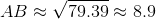# SAT II Math II : Other 2-Dimensional Geometry

## Example Questions

### Example Question #1 : Other 2 Dimensional Geometry

Which of the following describes a triangle with sides of length 9 feet, 4 yards, and 180 inches?

The triangle is acute and scalene.

The triangle is right and scalene.

The triangle is acute and isosceles, but not equilateral.

The triangle is right and isosceles, but not equilateral.

The triangle is acute and equilateral.

The triangle is right and scalene.

Explanation:

3 feet make a yard, so 9 feet is equal to 3 yards. 36 inches make a yard, so 180 inches is equal to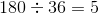yards. That makes this a 3-4-5 triangle. 3-4-5 is a well-known Pythagorean triple; that is, they have the relationship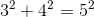and any triangle with these three sidelengths is a right triangle. Also, since the three sides are of different lengths, the triangle is scalene.

The correct response is that the triangle is right and scalene.

### Example Question #2 : Other 2 Dimensional Geometry

Which of the following describes a triangle with sides of length two yards, eight feet, and ten feet?

The triangle is right and scalene.

The triangle is right and isosceles.

The triangle is acute and scalene.

The triangle is acute and isosceles.

The triangle cannot exist.

The triangle is right and scalene.

Explanation:

Two yards is equal to six feet. The sidelengths are 6, 8, and 10, which form a well-known Pythagorean triple with the relationship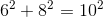The triangle is therefore right. Since no two sides have the same length, it is also scalene.

### Example Question #3 : Other 2 Dimensional Geometry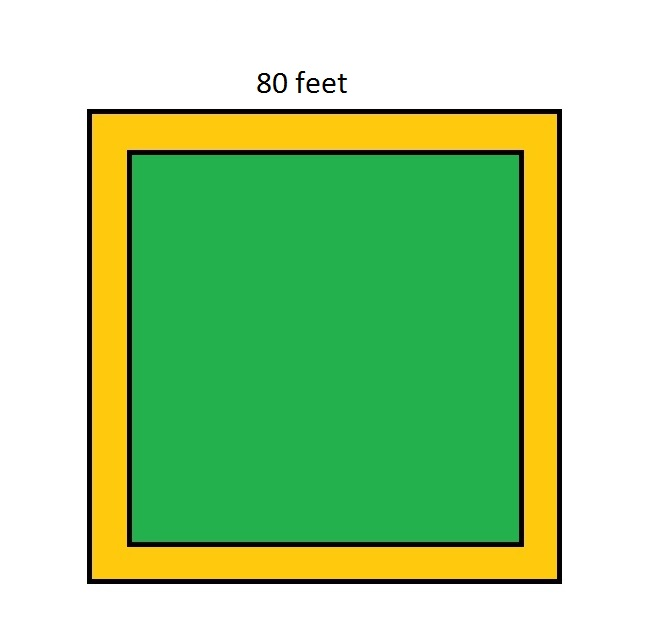The above figure shows a square garden (in green) surrounded by a dirt pathfeet wide throughout. Which of the following expressions gives the distance, in feet, from one corner of the garden to the opposite corner?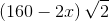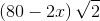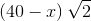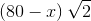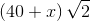Explanation:

The sidelength of the garden isfeet less than that of the entire lot - that is,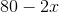. Since the garden is square, the path from one corner to the other is a diagonal of a square, which has lengthtimes the sidelength. This is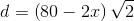feet.

### Example Question #4 : Other 2 Dimensional Geometry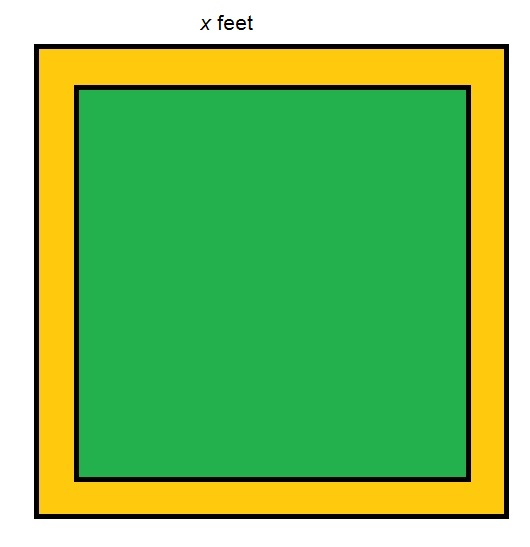The above figure shows a square garden (in green) surrounded by a dirt path six feet wide throughout. Which of the following expressions gives the distance, in feet, from one corner of the garden to the opposite corner?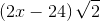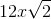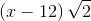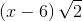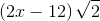Explanation:

The sidelength of the garden is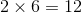less than that of the entire lot - that is,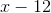. Since the garden is square, the path from one corner to the other is a diagonal of a square, which has lengthtimes the sidelength. This is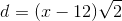feet.

### Example Question #5 : Other 2 Dimensional Geometry

A circle is inscribed inside a square that touches all edges of the square. The square has a length of 3.  What is the area of the region inside the square and outside the edge of the circle?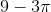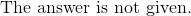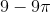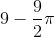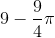Explanation:

Solve for the area of the square.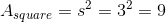Solve for the area of the circle.  Given the information that the circle touches all sides of the square, the diameter is equal to the side length of the square.

This means that the radius is half the length of the square: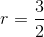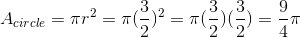Subtract the area of the square and the circle to determine the area desired.

The answer is:### Example Question #1 : Other 2 Dimensional Geometry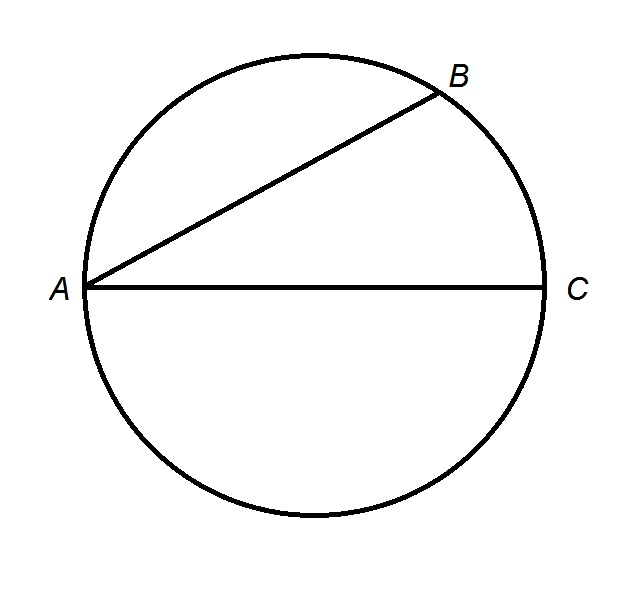Figure is not drawn to scale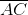is a diameter of the circle; its length is ten; furthermore we know the following: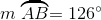Give the length of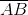(nearest tenth)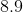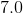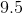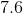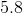Explanation:

Locate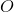, the center of the circle, which is the midpoint of; draw radius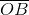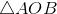is formed. The central angle that intercepts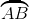is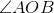, so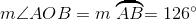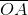and, being radii of the circle, have length half the diameter of ten, or five. The diagram is below.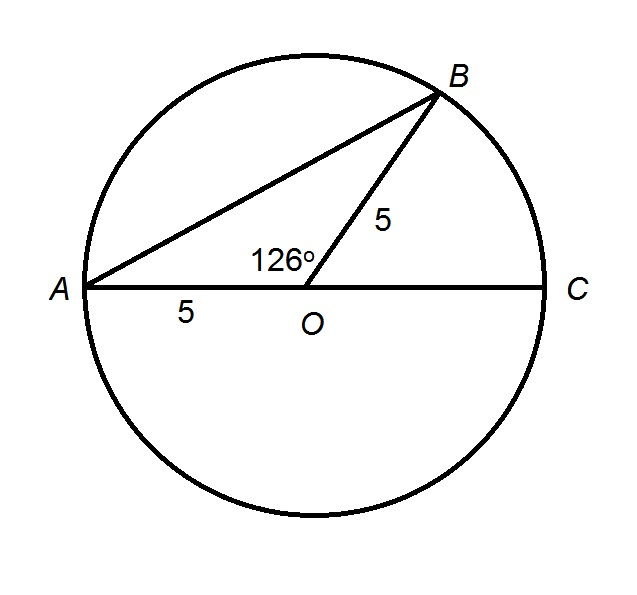By the Law of Cosines, given two sides of a triangle of lengthand, and their included angle of measure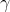, the length of the third sidecan be calculated using the formula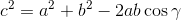Setting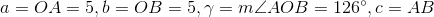, solve for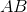: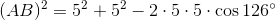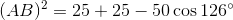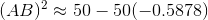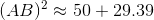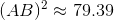Taking the square root of both sides: